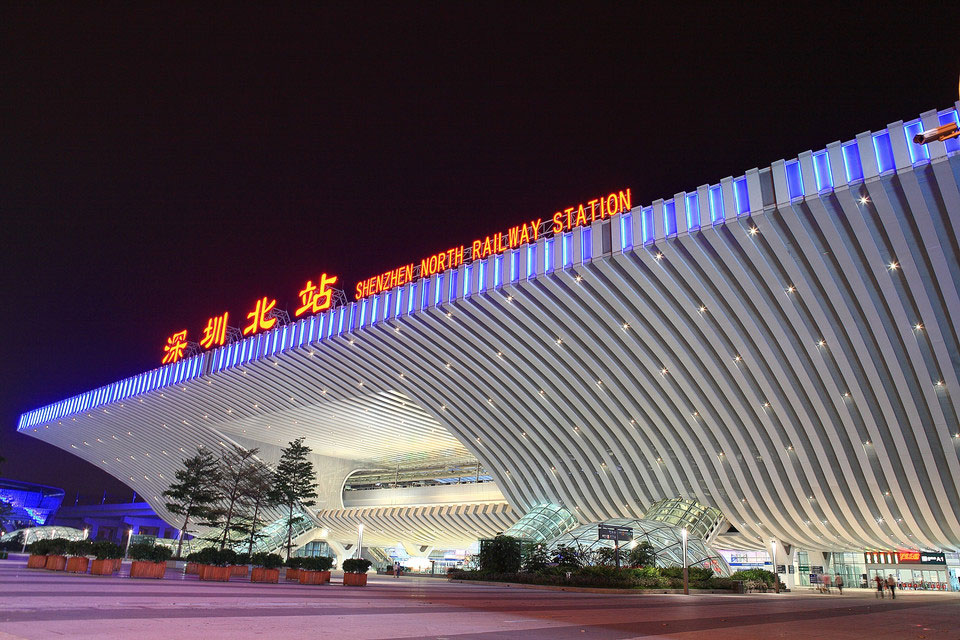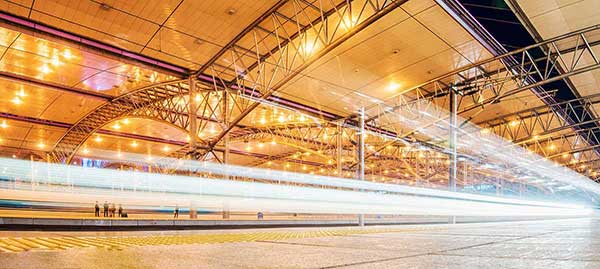•广州南站

可用休息室6个

可用餐厅2个

•深圳北站

可用休息室6个

可用餐厅0个

•长沙南站

可用休息室2个

可用餐厅0个

•重庆北站

可用休息室1个

可用餐厅0个

•西安北站

可用休息室1个

可用餐厅0个

•沈阳北站

可用休息室2个

可用餐厅0个

• 高铁列表
• 全部地点 中国大陆 其他地区

A

• 奥伦堡火车站俄罗斯联邦

B

• 北京南站

• 北京西站

• 北京站

• 璧山站

C

• 成都东站

• 长沙南站

HOT
• 重庆北站

HOT
• 重庆西站

• 车里雅宾斯火车站俄罗斯联邦

• 长春西站

D

• 大连站

• 大足南站

• 大连北站

• 垫江站

F

• 福州南站

• 福州站

• 福冈高铁博多站日本

G

• 广州南站

HOT
• 贵阳北站

• 桂林北站

• 桂林站

H

• 哈尔滨西站

• 杭州东站

• 合井站韩国

• 合肥站

• 合肥南站

• 杭州站

• 汉口站

J

• 简阳南站

• 济南西站

K

• 京都站日本

• 昆明南站

L

• 隆昌北站

• 兰州西站

• 罗马特米尼火车站意大利

• 柳州站

• 梁平南站

M

• 明洞站韩国

N

• 南宁站

• 南昌火车站

• 南昌西站

• 内江北站

• 宁波站

• 南京南站

• 南宁东站

• 南京站

O

• 大阪高铁站日本

Q

• 泉州站

• 青岛北站

• 秋明火车站俄罗斯联邦

R

• 荣昌北站

S

• 三亚站

• 深圳北站

HOT
• 上海站

• 沈阳北站

HOT
• 石家庄站

• 首尔站韩国

• 苏州北站

• 苏州站

• 上海虹桥站

• 沈阳站

• 沈阳站

• 上海西站

T

• 天津南站

• 天津西站

• 太原站

• 太原南站

• 唐山站

W

• 武汉站

• 温州南站

• 无锡站

• 无锡东站

• 无锡新区站

• 乌兰乌德火车站俄罗斯联邦

• 万州北站

X

• 西安北站

HOT
• 厦门站

• 徐州东站

• 厦门北站

• 新西伯利亚火车站俄罗斯联邦

Y

• 永川东站

• 盐城站

• 伊尔库茨克火车站俄罗斯联邦

• 叶卡捷琳堡火车站俄罗斯联邦

Z

• 珠海站

• 资阳北站

• 郑州东站

• 资中北站

A

B

C

D

F

G

H

J

K

L

M

N

O

Q

R

S

T

W

X

Y

Z